Home / Basic Electrical / Parallel RL Circuit

# Parallel RL Circuit

Want create site? Find Free WordPress Themes and plugins.

This guide covers Parallel RL Circuit Analysis, Phasor Diagram, Impedance & Power Triangle, and several solved examples along with the review questions answers.

The combination of a resistor and inductor connected in parallel to an AC source, as illustrated in Figure 1, is called a parallel RL circuit. In a parallel DC circuit, the voltage across each of the parallel branches is equal. This is also true of the AC parallel circuit.

The voltages across each parallel branch are:

• The same value.
• Equal in value to the total applied voltage ET.
• All in phase with each other.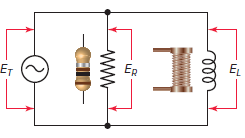Figure 1 Parallel RL circuit.

Therefore, for a RL parallel circuitn parallel DC circuits, the simple arithmetic sum of the individual branch currents equals the total current. The same is true in an AC parallel circuit if only pure resistors or only pure inductors are connected in parallel.

However, when a resistor and inductor are connected in parallel, the two currents will be out of phase with each other. In this case, the total current is equal to the vector sum rather than the arithmetic sum of the currents.

Recall that the voltage and current through a resistor are in phase, but through a pure inductor the current lags the voltage by exactly 90 degrees. This is still the case when the two are connected in parallel.

## Parallel RL Circuit Phasor Diagram

The relationship between the voltage and currents in a parallel RL circuit is illustrated in the vector (phasor) diagram of Figure 2 and summarized as follows:

• The reference vector is labeled E and represents the voltage in the circuit, which is common to all elements.
• Since the current through the resistor is in phase with the voltage across it, IR (2 A) is shown superimposed on the voltage vector.
• The inductor current IL (4 A) lags the voltage by 90 degrees and is positioned in a downward direction lagging the voltage vector by 90 degrees.
• The vector addition of IR and IL gives a resultant that represents the total (IT), or line current (4.5 A).
• The angle theta (θ) represents the phase between the applied line voltage and current.Figure 2 Parallel RL circuit vector (phasor) diagram.

As is the case in all parallel circuits, the current in each branch of a parallel RL circuit acts independent of the currents in the other branches. The current flow in each branch is determined by the voltage across that branch and the opposition to current flow, in the form of either resistance or inductive reactance, contained in the branch.

Ohm’s law can then be used to find the individual branch currents as follows: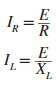The resistive branch current has the same phase as the applied voltage, but the inductive branch current lags the applied voltage by 90 degrees. As a result, the total line current (IT) consists of IR and IL 90 degrees out of phase with each other.

The current flow through the resistor and the inductor form the legs of a right triangle, and the total current is the hypotenuse. Therefore, the Pythagorean theorem can be applied to add these currents together by using the equation:In all parallel RL circuits, the phase angle theta (θ) by which the total current lags the voltage is somewhere between 0 and 90 degrees. The size of the angle is determined by whether there is more inductive current or resistive current.

If there is more inductive current, the phase angle will be closer to 90 degrees. It will be closer to 0 degrees if there is more resistive current. From the circuit vector diagram you can see that the value of the phase angle can be calculated from the equation: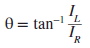Current in Parallel RL Circuit Example 1

For the parallel RL circuit shown in Figure 3, determine:

1. Current flow through the resistor.
2. Current flow through the inductor.
3. The total line current.
4. The phase angle between the voltage and total current flow.
5. Express all currents in polar notation.
6. Use a calculator to convert all currents to rectangular notation.Figure 3 Circuit for example 1.

Solution:

$\text{a}\text{. }{{\text{I}}_{\text{R}}}\text{=}\frac{\text{E}}{\text{R}}\text{=}\frac{\text{120V}}{\text{30 }\!\!\Omega\!\!\text{ }}\text{=4A}$

$\text{b}\text{. }{{\text{I}}_{\text{L}}}\text{=}\frac{\text{E}}{{{\text{X}}_{\text{L}}}}\text{=}\frac{\text{120V}}{\text{40 }\!\!\Omega\!\!\text{ }}\text{=3A}$

$\text{c}\text{. }{{\text{I}}_{\text{T}}}\text{=}\sqrt{\text{I}_{\text{R}}^{\text{2}}\text{+I}_{\text{L}}^{\text{2}}}\text{=}\sqrt{{{\text{4}}^{\text{2}}}\text{+}{{\text{3}}^{\text{2}}}}\text{=5A}$

$d.\theta ={{\tan }^{-1}}\left( \frac{{{I}_{L}}}{{{I}_{R}}} \right)={{\tan }^{-1}}\left( \frac{3}{4} \right)={{36.9}^{o}}$

$\begin{matrix}\text{e}\text{. }{{\text{I}}_{\text{T}}}\text{=5}\angle \text{-36}\text{.}{{\text{9}}^{\text{o}}} & {{\text{I}}_{\text{R}}}\text{=4}\angle {{\text{0}}^{\text{o}}} & {{\text{I}}_{\text{L}}}\text{=3}\angle \text{-9}{{\text{0}}^{\text{o}}} \\\end{matrix}$

$\text{f}\text{.}\begin{matrix}\text{ }{{\text{I}}_{\text{T}}}\text{=4-j3} & {{\text{I}}_{\text{R}}}\text{=4+j0} & {{\text{I}}_{\text{L}}}\text{=0-j3} \\\end{matrix}$

## Parallel RL Circuit Impedance

The impedance (Z) of a parallel RL circuit is the total opposition to the flow of current. It includes the opposition (R) offered by the resistive branch and the inductive reactance (XL) offered by the inductive branch.

The impedance of a parallel RL circuit is calculated similarly to a parallel resistive circuit. However, since XL and R are vector quantities, they must be added vectorially. As a result, the equation for the impedance of a parallel RL circuit consisting of a single resistor and inductor is:Where the quantity in the denominator is the vector sum of the resistance and inductive reactance. If there is more than one resistive or inductive branch, R and XL must equal the total resistance or reactance of theses parallel branches.

When the total current (IT) and the applied voltage are known, the impedance is more easily calculated using the Ohm’s law as follows:The impedance of a parallel RL circuit is always less than the resistance or inductive reactance of any one branch. This is because each branch creates a separate path for current flow, thus reducing the overall or total circuit opposition to the current flow.

The branch that has the greater amount of current flow (or lesser amount of opposition) has the most effect on the phase angle. This is the opposite of a series RL circuit. In a parallel RL circuit, if XL is larger than R, the resistive branch current is greater than the inductive branch current so the phase angle between the applied voltage and total current is closer to 0 degrees (more resistive in nature).

Impedance in Parallel RL Circuit Example 2

For the parallel RL circuit shown in Figure 4, determine:

1. Impedance (Z) based on the given R and XL values.
2. Current flow through the resistor and inductor.
3. The total line current.
4. Impedance (Z) based on the total current (IT) and the applied voltage values.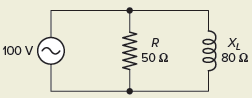Figure 4 Circuit for example 2.

Solution:

$Z=\frac{R{{X}_{L}}}{\sqrt{{{R}^{2}}+X_{L}^{2}}}=\frac{50\times 80}{\sqrt{{{50}^{2}}+{{80}^{2}}}}=42.4\Omega$

\begin{align}& {{I}_{R}}=\frac{E}{R}=\frac{100V}{50\Omega }=2A \\& {{I}_{L}}=\frac{E}{{{X}_{L}}}=\frac{100V}{80\Omega }=1.25A \\\end{align}

${{I}_{T}}=\sqrt{I_{R}^{2}+I_{L}^{2}}=\sqrt{{{2}^{2}}+{{1.25}^{2}}}=2.36A$

$Z=\frac{E}{{{I}_{T}}}=\frac{100V}{2.36A}=42.4\Omega$

## Power in Parallel RL Circuit

In the parallel RL circuit, the VA (apparent power) includes both the watts (true power) and the VARs (reactive power), as shown in Figure 5. The true power (W) is that power dissipated by the resistive branch, and the reactive power (VARs) is the power that is returned to the source by the inductive branch.

The relationship of VA, W, and VARs is the same for the RL parallel circuit as it is for the RL series circuit. The following is a summary of these formulas:

• The true power in watts is equal to the voltage drop across the resistor times the current flowing through it:• The reactive power in VARs is equal to the voltage drop across the inductor times the current flowing through it: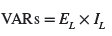• The apparent power in VA is equal to the applied voltage times the total current:Figure 5 Power components of a RL parallel circuit.

Figure 6 shows the power triangle for a RL parallel circuit. Apply the Pythagorean theorem, and the various power components can be determined using the following equations: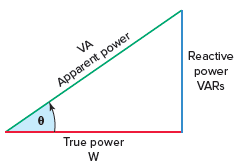Figure 6 Power triangle for a RL parallel circuit.

## Power Factor in Parallel RL Circuit

Power factor (PF) in a RL parallel circuit is the ratio of true power to the apparent power just as it is in the series RL circuit. There are, however, some differences in the other formulas used to calculate power factor in the series and parallel RL circuits.

In a series RL circuit, the power factor could be found by dividing the voltage drop across the resistor by the total applied voltage. In a parallel circuit the voltage is the same but the currents are different, and power factor can be calculated using the formulaAnother power factor formula that is different involves resistance and impedance. In the parallel RL circuit, the impedance will be less than the resistance. Therefore, when PF is computed using resistance and impedance, the formula used isParallel RL Circuit Calculations Example 3

For the parallel RL circuit shown in Figure 7, determine:

1. Current flow through the resistor.
2. True power in watts.
3. Current flow through the inductor.
4. Reactive power in VARs.
5. Inductance of the inductor.
6. Total current flow.
7. Circuit impedance.
8. Apparent power in VA.
9. Power factor.
10. The circuit phase angle θ.Figure 7 Circuit for example 3.

Solution:

• Step 1. Make a table and record all known values.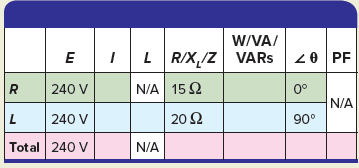• Step 2. Calculate the current through the resistor and enter the value in the table.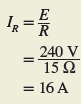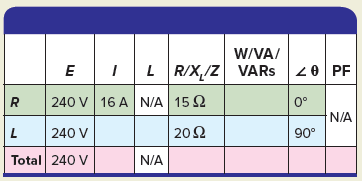• Step 3. Calculate the true power and enter the value in the table.• Step 4. Calculate the current through the inductor and enter the value in the table.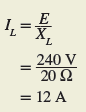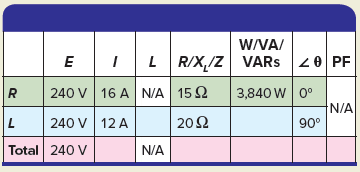• Step 5. Calculate the reactive power and enter the value in the table.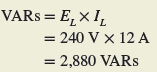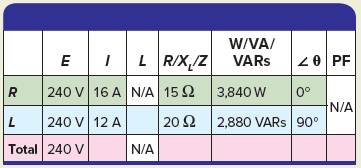• Step 6. Calculate the inductance of the inductor and enter the value in the table.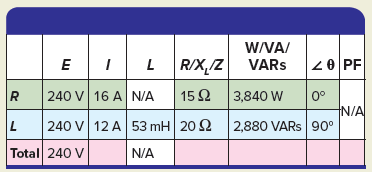• Step 7. Calculate the total current and enter the value in the table.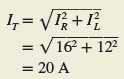• Step 8. Calculate the impedance and enter the value in the table.• Step 9. Calculate the apparent power and enter the value in the table.• Step 10. Calculate the power factor and enter the value in the table.• Step 11. Calculate the circuit phase angle θ and enter the value in the table.Review Questions

1. List three characteristics of the voltage across each branch of a parallel RL circuit.
2. In a parallel RL circuit the total current is equal to the vector sum rather than the arithmetic sum. Why?
3. What is used as the reference vector in the vector diagram of a parallel RL circuit?
4. Assume the resistive element of a parallel RL circuit is increased. Will this cause the phase angle of the circuit to increase or decrease? Why?
5. In a parallel RL circuit the impedance or total opposition is always less than that of the individual resistance or inductive reactance. Why?
6. Define the terms apparent power, reactive power, and true power as they apply to the parallel RL circuit.
7. Current measurements of a parallel RL circuit indicate a current flow of 2 amperes through the resistive branch and 4 amperes through the inductive branch. Determine:
1. The value of the total current flow.
2. The phase angle between the voltage and total current.
8. For the parallel RL circuit shown in Figure 8, determine:
1. Apparent power.
2. True power.
3. Reactive power.
4. Circuit power factor.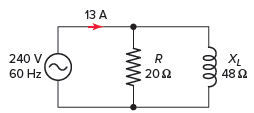Figure 8 Circuit for review question 8.

9. Complete a table for all given and unknown quantities for the parallel RL circuit shown in Figure 9.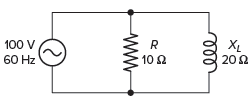Figure 9 Circuit for review question 9.

1. The voltage across each branch of a parallel RL circuit is the same value, equal in value to the total applied voltage, ET, and in phase with each other.
2. The total current in a parallel RL circuit is equal to the vector sum of the branch currents because the branch currents are out of phase with each other.
3. The reference vector in a parallel RL circuit is the applied voltage E.
4. If the resistive element of a parallel RL circuit is increased the resistive current will be decreased and the phase angle will be increased because the circuit is now more inductive.
5. Each branch creates a separate path for current flow thus acting to reduce the overall or total circuit resistance.
6. In the parallel RL circuit, the VA (apparent power) includes both the Watts (true power) and the VARs (reactive power), the true power (Watts) is that power dissipated by the resistive branch, and the reactive power (VARs) is the power that is returned to the source by the inductive branch.
7. (a) 4.47 A, (b) 63.4°
8. (a) 3120 VA, (b) 2880 W, (c) 1200 VARs, (d) 92.3% lagging
 E I R /XL /Z W/VA/VARs PF R 100 V 10 A 10 Ω 1000 W 0 L 100 V 5 A 20 Ω 500 VARs 90 Total 100 V 11.2 A 8.93 Ω 1120 VA 26.8 89.3%
Did you find apk for android? You can find new Free Android Games and apps.